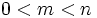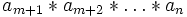# Associative implies generalized associative

## Statement

Let$S$ be a set and$*$ be a binary operation on$S$. Suppose that$*$ is an associative binary operation; in other words:$a * (b * c) = (a * b) * c \ \forall \ a,b,c \in S$

Then, for any positive integer$n$, every possible parenthesization of the expression:$a_1 * a_2 * \dots * a_n$

is equivalent.

## Proof

The technique of proof here is a strong form of induction: we assume the result is true for every$m < n$, and we prove it for$n$. Note that the cases$n = 1,2$ are tautological. For the case$n = 3$, there are precisely two possible parenthesizations, and associativity tells us that they are both equal.

Thus, assume$n > 3$, and that the result holds for all$m < n$. We will show that any way of parenthesization of:$a_1 * a_2 * \dots * a_n$

is equal to the left-associated expression:$((\dots ( a_1 * a_2) * a_3) * \dots ) * a_n)$

Let's prove this. For any method of parenthesization, there is an outermost multiplication, and in terms of this outermost multiplication, we can view the parenthesization as:$A * B$

where$A = a_1 * \dots * a_m$ and$B = a_{m+1} * \dots * a_n$, both parenthesized in some unknown way, with$0 < m < n$. Applying the induction assumption,$A$ equals the left-associated expression for$a_1 * a_2 * \dots * a_m$ and$B$ equals the left-associated expression for$a_{m+1} * a_{m+2} * \dots * a_n$.

Now, in the case that$B$ is a single letter (i.e.,$m = n - 1$), we are already done:$A * B$ equals the left-associated expression. If not, we can write$A * B$ as:$A * (C * a_n)$

where$C$ is the left-associated expression for$a_{m+1} * \dots * a_{n-1}$. Applying associativity, we get that the original expression equals:$(A * C) * a_n$

which is precisely the left-associated expression for$a_1 * a_2 * \dots * a_n$.